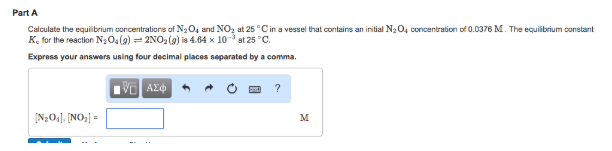# Problem: Calculate the equilibrium concentrations of N2O4 and NO2 at 25°C in a vessel that contains an initial N2O4 concentration of 0.0376 M The equilibrium constant Kc for the reaction N2O4(g) ⇌ 2NO2(g) is 4.64 x 10-3 at 25°C.

###### FREE Expert Solution
81% (298 ratings)###### Problem Details

Calculate the equilibrium concentrations of N2O4 and NO2 at 25°C in a vessel that contains an initial N2O4 concentration of 0.0376 M The equilibrium constant Kc for the reaction N2O4(g) ⇌ 2NO2(g) is 4.64 x 10-3 at 25°C.